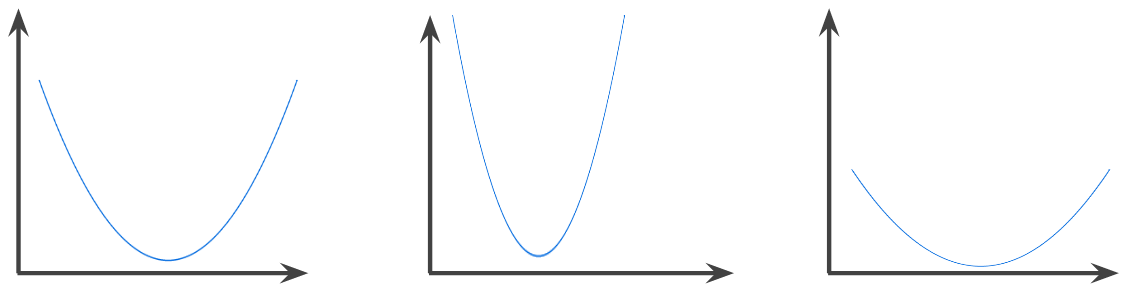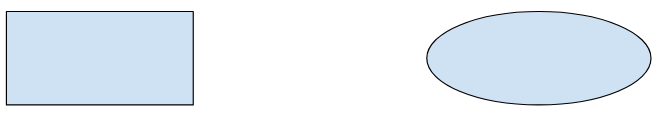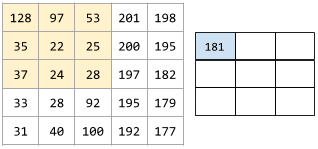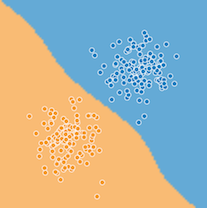# 机器学习术语表

## 准确率 (accuracy)

$$\text{准确率} = \frac{\text{正确的预测数}} {\text{样本总数}}$$

$$\text{准确率} = \frac{\text{正例数} + \text{负例数}} {\text{样本总数}}$$

## ROC 曲线下面积 (AUC, Area under the ROC Curve)

ROC 曲线下面积是，对于随机选择的正类别样本确实为正类别，以及随机选择的负类别样本为正类别，分类器更确信前者的概率。

## 偏差 (bias)

$$y' = b + w_1x_1 + w_2x_2 + … w_nx_n$$

## 聚类 (clustering)## 凸函数 (convex function)## 凸集 (convex set)## 卷积层 (convolutional layer)• 卷积层
• 池化层
• 密集层

## 卷积运算 (convolutional operation)

1. 卷积过滤器和输入矩阵切片执行元素级乘法。（输入矩阵切片与卷积过滤器具有相同的等级和大小。）
2. 对生成的积矩阵中的所有值求和。## 决策边界 (decision boundary)## 嵌套 (embeddings)

• 表示成包含百万个元素（高维度）的稀疏向量，其中所有元素都是整数。向量中的每个单元格都表示一个单独的英文单词，单元格中的值表示相应单词在句子中出现的次数。由于单个英文句子包含的单词不太可能超过 50 个，因此向量中几乎每个单元格都包含 0。少数非 0 的单元格中将包含一个非常小的整数（通常为 1），该整数表示相应单词在句子中出现的次数。
• 表示成包含数百个元素（低维度）的密集向量，其中每个元素都存储一个介于 0 到 1 之间的浮点值。这就是一种嵌套。

• 不同的初始化
• 不同的超参数
• 不同的整体结构

## Estimator

tf.Estimator 类的一个实例，用于封装负责构建 TensorFlow 图并运行 TensorFlow 会话的逻辑。您可以创建自定义 Estimator（如需相关介绍，请点击此处），也可以实例化其他人预创建的 Estimator

## 假正例率（false positive rate, 简称 FP 率）

ROC 曲线中的 x 轴。FP 率的定义如下：

$$\text{假正例率} = \frac{\text{假正例数}}{\text{假正例数} + \text{负例数}}$$

## 特征列 (tf.feature_column)

“特征列”是 Google 专用的术语。特征列在 Yahoo/Microsoft 使用的 VW 系统中称为“命名空间”，也称为

## 特征规范 (feature spec)

• 要提取的数据（即特征的键）
• 数据类型（例如 float 或 int）
• 长度（固定或可变）

Estimator API 提供了一些可用来根据给定 FeatureColumns 列表生成特征规范的工具。

## 广义线性模型 (generalized linear model)

• 最优的最小二乘回归模型的平均预测结果等于训练数据的平均标签。
• 最优的逻辑回归模型预测的平均概率等于训练数据的平均标签。

## 图 (graph)

TensorFlow 中的一种计算规范。图中的节点表示操作。边缘具有方向，表示将某项操作的结果（一个张量）作为一个操作数传递给另一项操作。可以使用 TensorBoard 直观呈现图。

## 合页损失函数 (hinge loss)

$$\text{loss} = \text{max}(0, 1 - (y' * y))$$

$$y' = b + w_1x_1 + w_2x_2 + … w_nx_n$$

“y”表示真标签，值为 -1 或 +1。## k-means

• 以迭代方式确定最佳的 k 中心点（称为形心）。
• 将每个样本分配到最近的形心。与同一个形心距离最近的样本属于同一个组。

k-means 算法会挑选形心位置，以最大限度地减小每个样本与其最接近形心之间的距离的累积平方。## k-median

k-means 紧密相关的聚类算法。两者的实际区别如下：

• 对于 k-means，确定形心的方法是，最大限度地减小候选形心与它的每个样本之间的距离平方和。
• 对于 k-median，确定形心的方法是，最大限度地减小候选形心与它的每个样本之间的距离总和。

• k-means 采用从形心到样本的欧几里得距离。（在二维空间中，欧几里得距离即使用勾股定理来计算斜边。）例如，(2,2) 与 (5,-2) 之间的 k-means 距离为：
$${\text{欧几里德距离}} = {\sqrt {(2-5)^2 + (2--2)^2}} = 5$$
• k-median 采用从形心到样本的曼哈顿距离。这个距离是每个维度中绝对差异值的总和。例如，(2,2) 与 (5,-2) 之间的 k-median 距离为：
$${\text{曼哈顿距离}} = \lvert 2-5 \rvert + \lvert 2--2 \rvert = 7$$

## lambda

（多含义术语，我们在此关注的是该术语在正则化中的定义。）

## Layers API (tf.layers)

• 通过 tf.layers.Dense 构建全连接层
• 通过 tf.layers.Conv2D 构建卷积层。

Layers API 遵循 Keras layers API 规范。也就是说，除了前缀不同以外，Layers API 中的所有函数均与 Keras layers API 中的对应函数具有相同的名称和签名。

## 对数几率 (log-odds)

$${\text{几率}} = \frac{\text{p}} {\text{(1-p)}} = \frac{.9} {.1} = {\text{9}}$$

$${\text{对数几率}} = ln(9) ~= 2.2$$

## 模型 (model)

• 一种 TensorFlow 图，用于表示预测的计算结构。
• 该 TensorFlow 图的特定权重和偏差，通过训练决定。

## 模型函数 (model function)

Estimator 中的函数，用于实现机器学习训练、评估和推断。例如，模型函数的训练部分可以处理以下任务：定义深度神经网络的拓扑并确定其优化器函数。如果使用预创建的 Estimator，则有人已为您编写了模型函数。如果使用自定义 Estimator，则必须自行编写模型函数。

NaN 是“非数字”的缩写。

• 一个元素设为 1。
• 所有其他元素均设为 0。

• 动物和非动物
• 蔬菜和非蔬菜
• 矿物和非矿物

## 操作 (op, Operation)

TensorFlow 图中的节点。在 TensorFlow 中，任何创建、操纵或销毁张量的过程都属于操作。例如，矩阵相乘就是一种操作，该操作以两个张量作为输入，并生成一个张量作为输出。

## 优化器 (optimizer)

• 动量 (Momentum)
• 稀疏性/正则化 (Ftrl)
• 更复杂的数学方法（Proximal，等等）

## 离群值 (outlier)

• 绝对值很高的权重
• 与实际值相差很大的预测值。
• 值比平均值高大约 3 个标准偏差的输入数据。

## 性能 (performance)

• 在软件工程中的传统含义。即：相应软件的运行速度有多快（或有多高效）？
• 在机器学习中的含义。在机器学习领域，性能旨在回答以下问题：相应模型的准确度有多高？即模型在预测方面的表现有多好？

## 困惑度 (perplexity)

$$P= 2^{-\text{cross entropy}}$$

## 池化 (pooling)## 精确率 (precision)

$$\text{精确率} = \frac{\text{正例数}} {\text{正例数} + \text{假正例数}}$$

## 等级 (rank)

• 张量中的维数。例如，标量等级为 0，向量等级为 1，矩阵等级为 2。
• 在将类别从最高到最低进行排序的机器学习问题中，类别的顺序位置。例如，行为排序系统可以将狗狗的奖励从最高（牛排）到最低（枯萎的羽衣甘蓝）进行排序。

## 召回率 (recall)

$$\text{召回率} = \frac{\text{正例数}} {\text{正例数} + \text{假负例数}}$$

## 修正线性单元 (ReLU, Rectified Linear Unit)

• 如果输入为负数或 0，则输出 0。
• 如果输入为正数，则输出等于输入。

## 正则化率 (regularization rate)

$$\text{最小化(损失方程 + }\lambda\text{(正则化方程))}$$

## S 型函数 (sigmoid function)

$$y = \frac{1}{1 + e^{-\sigma}}$$

$$\sigma = b + w_1x_1 + w_2x_2 + … w_nx_n$$

## 稀疏表示法 (sparse representation)

• 要采用密集表示法来表示此句子，则必须为所有一百万个单元格设置一个整数，然后在大部分单元格中放入 0，在少数单元格中放入一个非常小的整数。
• 要采用稀疏表示法来表示此句子，则仅存储象征句子中实际存在的单词的单元格。因此，如果句子只包含 20 个独一无二的单词，那么该句子的稀疏表示法将仅在 20 个单元格中存储一个整数。

0 a 0
1 aardvark 0
2 aargh 0
3 aarti 0
… 出现次数为 0 的另外 140391 个单词
140395 dogs 1
… 出现次数为 0 的 633062 个单词
773458 tails 1
… 出现次数为 0 的 189136 个单词
962594 wag 1
… 出现次数为 0 的很多其他单词

140395 dogs 1
773458 tails 1
962594 wag 1

## 稀疏性 (sparsity)

$${\text{稀疏性}} = \frac{\text{98}} {\text{100}} = {\text{0.98}}$$

## 结构风险最小化 (SRM, structural risk minimization)

• 期望构建最具预测性的模型（例如损失最低）。
• 期望使模型尽可能简单（例如强大的正则化）。

## 步长 (stride)## 合成特征 (synthetic feature)

• 对连续特征进行分桶，以分为多个区间分箱。
• 将一个特征值与其他特征值或其本身相乘（或相除）。
• 创建一个特征组合

## 张量 (Tensor)

TensorFlow 程序中的主要数据结构。张量是 N 维（其中 N 可能非常大）数据结构，最常见的是标量、向量或矩阵。张量的元素可以包含整数值、浮点值或字符串值。

## 正例率（true positive rate, 简称 TP 率）

$$\text{正例率} = \frac{\text{正例数}} {\text{正例数} + \text{假负例数}}$$

## 宽度模型 (wide model)

[{ "type": "thumb-down", "id": "missingTheInformationINeed", "label":"没有我需要的信息" },{ "type": "thumb-down", "id": "tooComplicatedTooManySteps", "label":"太复杂/步骤太多" },{ "type": "thumb-down", "id": "outOfDate", "label":"内容需要更新" },{ "type": "thumb-down", "id": "translationIssue", "label":"翻译问题" },{ "type": "thumb-down", "id": "samplesCodeIssue", "label":"示例/代码问题" },{ "type": "thumb-down", "id": "otherDown", "label":"其他" }]
[{ "type": "thumb-up", "id": "easyToUnderstand", "label":"易于理解" },{ "type": "thumb-up", "id": "solvedMyProblem", "label":"解决了我的问题" },{ "type": "thumb-up", "id": "otherUp", "label":"其他" }]